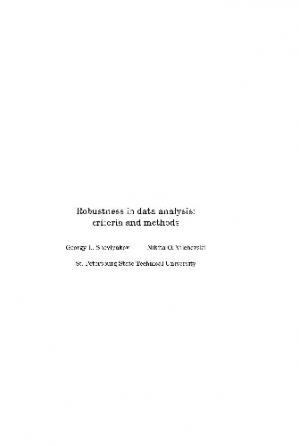## Change Language# Robustness in data analysisAuthor(s): Georgy L. Shevlyakov, Nikita O. Vilchevski Collection: Modern Probability and Statistics, 6 Publisher: Walter de Gruyter Year: 2001 Language: English Pages: 320 pages Size: 1.98 MB Extension: PDF

[tab] [content title="Description"]The field of mathematical statistics called robustness statistics deals with the stability of statistical inference under variations of accepted distribution models. Although robustness statistics involves mathematically highly defined tools, robust methods exhibit a satisfactory behaviour in small samples, thus being quite useful in applications. This volume addresses various topics in the field of robust statistics and data analysis, such as: a probability-free approach in data analysis; minimax variance estimators of location, scale, regression, autoregression and correlation; L1-norm methods; adaptive, data reduction, bivariate boxplot, and multivariate outlier detection algorithms; applications in reliability, detection of signals, and analysis of the sudden cardiac death risk factors. The text contains results related to robustness and data analysis technologies, including both theoretical aspects and practical needs of data processing. [/content] [content title="Content"] [/content] [content title="About the author"]Georgy Leonidovich Shevlyakov, Russian mathematics professor, researcher. Member of Institute of Electrical and Electronics Engineers. [/content] [/tab]